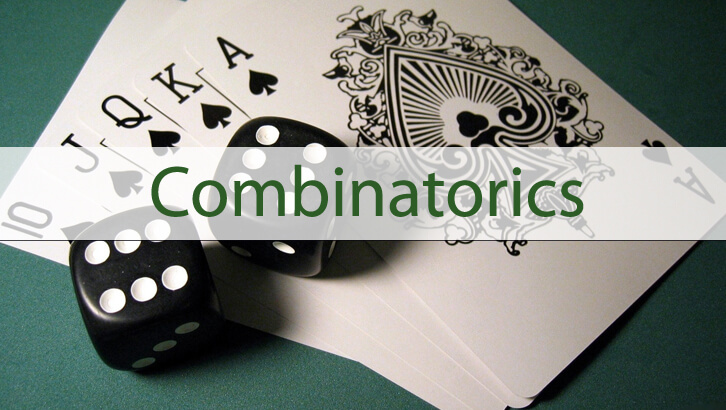# Questions and solutions on Permutation and Combination

Permutation and Combination.

Questions
1. Sum of three natural numbers a, b and c is 10. How many ordered triplets (a, b, c) exist?

2. In how many ways 11 identical toys be placed in 3 distinct boxes such that no box is empty?

Correct Answers:
1. 9C2
2. 10C2

Explanatory Answers

1. Sum of three natural numbers a, b and c is 10. How many ordered triplets (a, b, c) exist?

a + b + c = 10. Now, let us place ten sticks in a row

|     |     |     |    |     |     |     |     |     |

This question now becomes the equivalent of placing two ‘+’ symbols somewhere between these sticks. For instance

|     |     |     | + |     |     |     |     |  + |, This would be the equivalent of 4 + 5 + 1. or, a = 4, b = 5, c = 1.

There are 9 slots between the sticks, out of which one has to select 2 for placing the ‘+’s.

The number of ways of doing this would be 9C2. Bear in mind that this kind of calculation counts ordered triplets. (4,5,1) and (1, 4, 5) will both be counted as distinct possibilities.

We can also do a + b + c = n where a, b, c have to be whole numbers (instead of natural numbers as in this question) with a small change to the above approach. Give it some thought.

2. In how many ways 11 identical toys be placed in 3 distinct boxes such that no box is empty?
This is nothing but number of ways of having a,b,c such that a + b + c = 11, where a, b, c are natural numbers.

10C2.

The two questions stated above are almost identical ones.

CAT 2013 Course offered by 2IIM @ ChennaiCourse handled by IIM Alumni, Batches @ Anna Nagar, Mylapore and Velachery. Weekend batches @ Anna Nagar from 24th November, @ Mylapore from 10th November, @ Velachery from 17th November.